# Beginners Guide to Data Visualization

December 29th, 2020Data Visualization is the art of representing data in the form of charts and graphics. We’ve all heard visualizations are essential, but why are they important exactly?

Visual representations of data allow you to better understand data’s underlying pattern. Human eyes are automatically drawn towards colors and patterns. Therefore, when we look at the data in the form of a graph or a chart, we immediately notice what the data is trying to tell us. Let us take a look at the “Iris” dataset, if the data is presented row-wise to you as shown below, it is very difficult to understand how many types of flowers are in it unless you spend a copious amount of time going through the dataset row by row.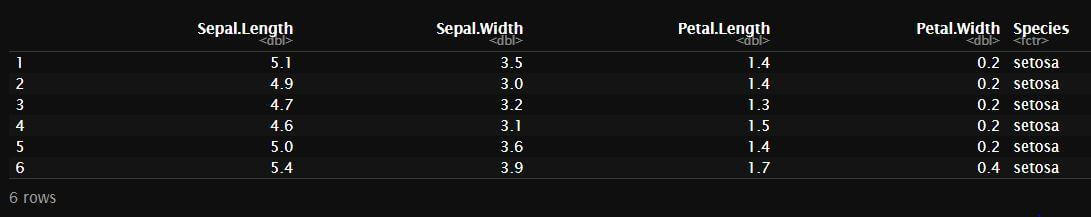But if the same dataset is presented as a plot as shown below, it is easier to figure out how many types of flowers are in the dataset, based on the colors of the data points.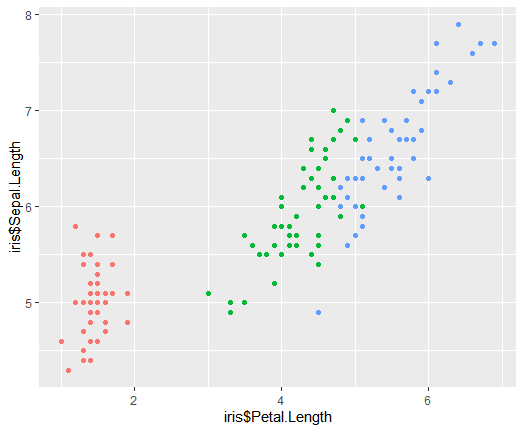Representing the data as graphs and charts tell us a far better story than the old row-wise format does. In the age of Big Data, visualization is an essential tool in understanding and making sense of the humongous amount of data. The visuals tell us a story by highlighting the trends and outliers, separating the noise from useful data, and presenting the results of our analysis in a simple but powerful way. In Data Science, data visualization is a key part of Exploratory Data Analysis, Data Mining, and presenting the results of a model. Although underplayed, this tool is a must in every data scientist and ML engineer’s ever-growing toolkit.

As much as the graphics are important, it is of no use if it does not tell a good story. For example, if the graph is too bland, it will not catch any attention, if it is too simple, it might not tell you all that you want to know about the data, if it is too complicated and overdone, it might just be too much to gain any clarity. There needs to be a proper combination of the data and the visuals presented.

As mentioned before, a graph is of no use if it does not represent the data well, but how do you make sure that your graphic will catch the viewer’s attention? Start with the right kind of graph, there are many different types of graphs and charts you can make for a given dataset, but to make sure it conveys the right story, it is important to choose the graph that fits well with the type of data you have and the analysis done.

Types of Graphs:

Although there are many types of graphs, charts, and maps used, these are some that you as a data scientist must-have in your toolkit:

• Bar Graph
• Histogram
• Scatter Plot
• Box Plot
• Line graph
• Pie Chart

Now let’s go over each of these graph and chart types and see what they are, when they can be used, and when not to be used.

Bar Graph: Bar graph is one of the simplest ways to present data with clarity, it is normally used to compare single variable values among different categories. You can present your bar graphs either horizontally or vertically. Bar graphs can also be used to track the trend of few variables over time. The length of each bar represents the value of the variables, upon seeing the graph below, it is easy to compare each of those models according to their Adjusted R^2 values.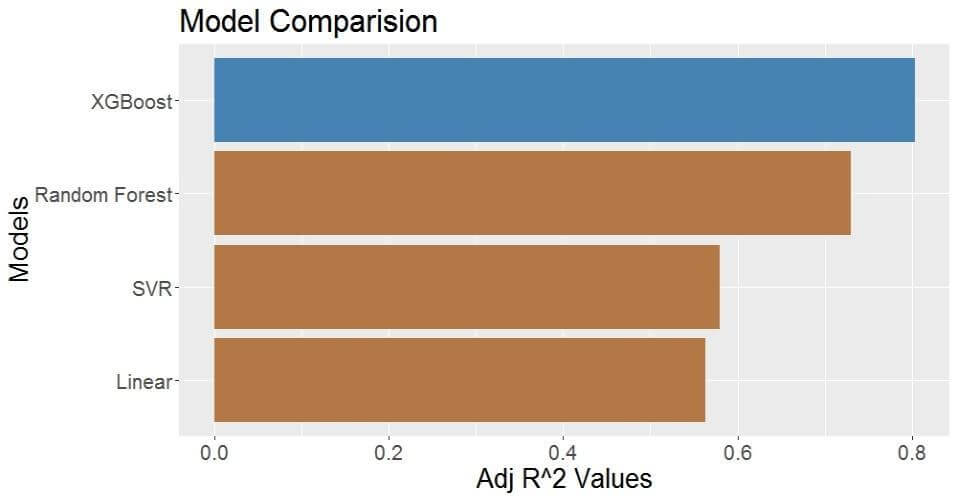When to use Bar Graph:

• Comparing a few variables of the same category
• Comparing 2 categorical variables
• Comparing Categorical vs Continuous variables
•

When not to use bar graph:

• Comparing a large number of categories
• Comparing continuous vs continuous data

Histogram: Although a histogram looks quite similar to a bar graph, it serves a different purpose. A histogram represents the distribution of numeric data. It breaks data into intervals called bins and counts the occurrence of each data point in that bin. On the x-axis, you have your bins, and on the y-axis the count of occurrences of data points in each of those bins. The number of bins can be set by the user, so you have to make sure the number of bins set is neither too small nor too big, that way the histogram presents the data.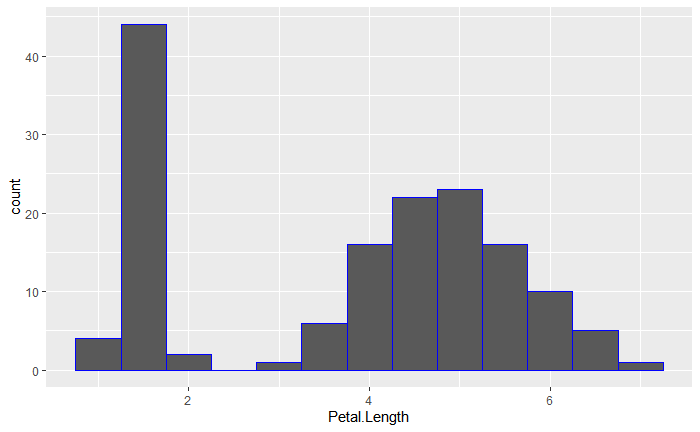When to use Histograms:

• Data is numerical/continuous
• Determining the shape of the distribution of the data
•

When not to use Histograms:

• Data is categorical

Scatter Plot: Scatter plots show individual data points, usually it is used to determine the relationship between two variables. One axis measures one variable and the other one measures a different variable. Scatter plots can also be used to show correlations or cluster patterns in the data. In addition to these, scatter plots also make it easy to identify the outliers. The scatter plot shown below shows us the Petal Length of flowers in the Iris dataset vs the Petal Width, we can immediately notice the clusters in the graph.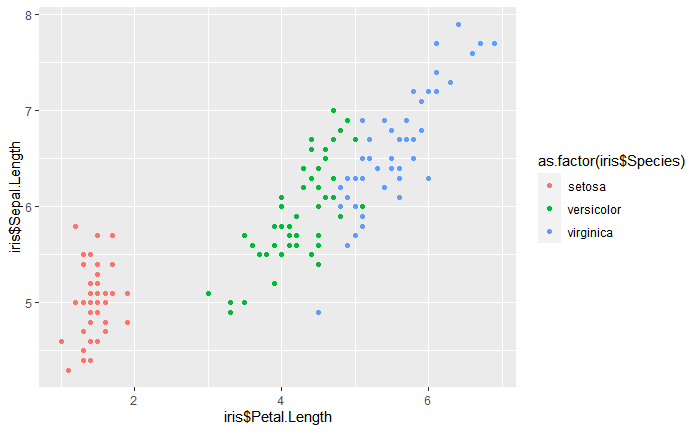• Analyzing individual points
• Outlier analysis
• Understanding variable relationships
• Analysis of data distribution
• Data is continuous
•

When not to use Scatter Plots:

• Working with 1-dimensional data
• Looking for precision
• Data is categorical
•

Box Plot: Box plot, sometimes also called Box and Whisker plot presents a 5 point summary of the data. As the name suggests it usually presents the data as a box with some lines in it that represent, the maximum value, third quartile, median, first quartile, and the minimum, in addition to these the box plot also plots any outliers in the data.

The maximum and minimum lines are the maximum and the minimum values from the dataset. The median line representing the median of the dataset separates the higher half of the data from the lower half. The third quartile line represents the median of the upper half of the data and the first quartile the median of the lower half. The box plot also shows us the IQR- Inter Quartile Range, which gives us the middle 50% of the data, IQR range is between the third quartile and first quartile lines. In addition to representing all this data, box plots also tell us if the data is symmetrical, how tightly is the data grouped, if, and how is it skewed. Box plot, although not as fancy as the other graphs, is powerful as it can present all this data in a small space and allows you to compare multiple variables using multiple sides by side box plots.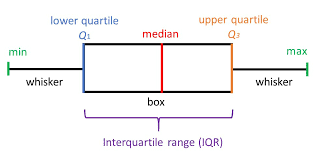When to use Box plots:

• Analyzing or Comparing data distribution over multiple series
• Working with categorical vs continuous data
•

When not to use Box plots:

• Working with a small dataset
• Looking for precision
• Working with categorical vs categorical data

Line Graph: Line graph is similar to a scatter plot, but instead of displaying just the data points, as the name suggests, the line graph connects those points with a line. It is usually used to analyze a trend in data over intervals of time. Line graphs are drawn between 2 variables where the independent variable is plotted on the x-axis and the dependent variable is plotted on the y-axis. It allows you to plot multiple trends in the same graph to compare.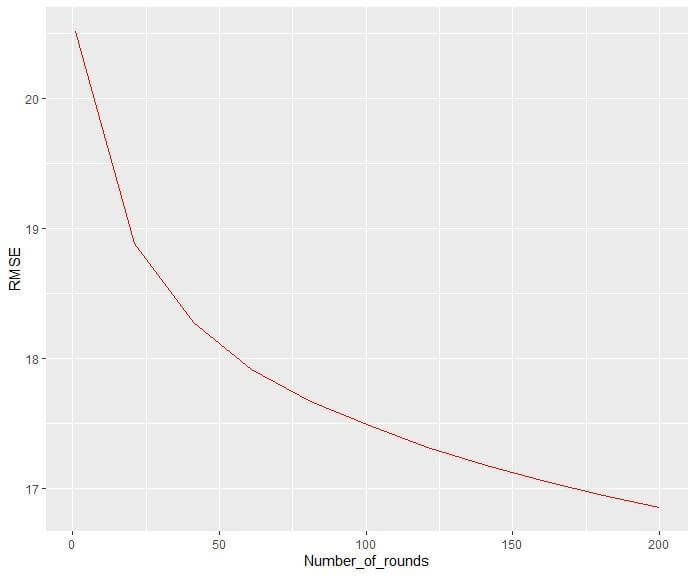When to use a Line graph:

• Working with Continuous data
• Analyzing trends
• Predicting future values
•

When not to use Line graphs:

• Trying to get a general overview of the data
• Analyzing individual components

Pie Chart: Pie chart is a circular plot that is divided into sections that represent the numerical proportions. It looks sort of like a cake sliced into pieces. Although not widely used, it might be useful in many scenarios. The pie chart usually presents parts of the whole data and is plotted to show the composition of static data.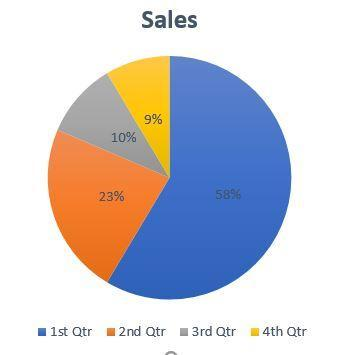When to use Pie Charts:

• Comparing relative values or parts of the whole
• Working with Static data
•

When not to use Pie Charts:

• The parts do not add up to a whole
• Data requires you to make a large number of slices

These are some of the commonly used graphs in data science, but by no means is this list exhaustive. Some other types of graphs you can check out are:

• Stacked Bar graph
• Heat Maps
• Area Chart
• Tree Map
• Bubble Chart
• Butterfly Plot
• Funnel Charts

The list goes on.

Summary

Data visualization is an important part of data science. Human eyes are best at deciphering the hidden patterns, trends, and in general understanding data better when it is presented in an eye-catching visual. Not only does data visualization helps in understanding the data better, but it also helps us tell a visual story for the analysis done upon the data. We saw 6 different types of graphics and when to use them and when not to use them and also what each graph can tell us. Using the right types of graphics, data can be understood and presented with clarity.Manaswini is a graduate student of M.S in Data Science from Northeastern University. She is a Bioinformatics Analytics intern at EMD Serono, Billerica and President of Data Science Hub at Northeastern University.

2

## Alumni SpeaksSrivatsDivya